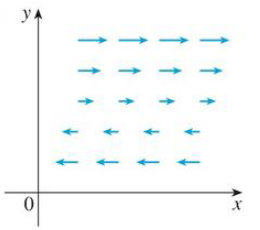Chapter 16.5, Problem 11E

Chapter
Section
Textbook Problem

The vector field F is shown in the xy-plane and looks the same in all other horizontal planes. (In other words, F is independent of z and its z-component is 0.)(a) Is div F positive, negative, or zero? Explain.(b) Determine whether curl F = 0. If not, in which direction does curl F point?11.(a)

To determine

To find and explain: The div F.

Explanation

Formula used:

Consider the standard equation of a divergence of vector field for F=Pi+Qj+Rk

divF=Px+Qy+Rz (1)

As F is independent of z-component (R=0) is Rx=Ry=Rz=0

(b)

To determine

Whether curl F=0 or not.

Still sussing out bartleby?

Check out a sample textbook solution.

See a sample solution

The Solution to Your Study Problems

Bartleby provides explanations to thousands of textbook problems written by our experts, many with advanced degrees!

Get Started

Expand each expression in Exercises 122. (3x+1)(2x2x+1)

Finite Mathematics and Applied Calculus (MindTap Course List)

|2x 3| 5

Applied Calculus for the Managerial, Life, and Social Sciences: A Brief Approach

2. True or false: (a) (b) (c) (d) (e) To evaluate , our first step to divide into 4x.

Mathematical Applications for the Management, Life, and Social Sciences

23. Let where . Prove .

Elements Of Modern Algebra

2 1 0 does not exist

Study Guide for Stewart's Multivariable Calculus, 8th

Sometime, Always, or Never:

Study Guide for Stewart's Single Variable Calculus: Early Transcendentals, 8th